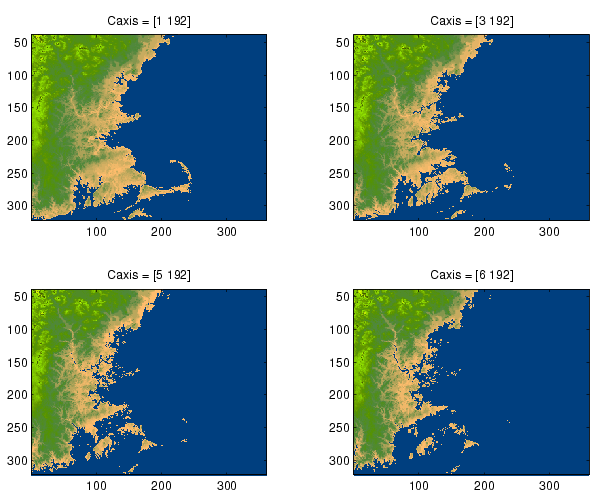MATLAB Function Referencecaxis

Color axis scaling

Syntax

• ```caxis([cmin cmax])
caxis auto
caxis manual
caxis(caxis)
v = caxis
caxis(axes_handle,...)
```

Description

`caxis` controls the mapping of data values to the colormap. It affects any surfaces, patches, and images with indexed CData and CDataMapping set to scaled. It does not affect surfaces, patches, or images with true color CData or with CDataMapping set to direct.

```caxis([cmin cmax]) ``` sets the color limits to specified minimum and maximum values. Data values less than `cmin` or greater than `cmax` map to `cmin` and `cmax`, respectively. Values between `cmin` and `cmax` linearly map to the current colormap.

```caxis auto ``` lets MATLAB compute the color limits automatically using the minimum and maximum data values. This is the default behavior. Color values set to `Inf` map to the maximum color, and values set to -`Inf` map to the minimum color. Faces or edges with color values set to `NaN` are not drawn.

```caxis manual and caxis(caxis) ``` freeze the color axis scaling at the current limits. This enables subsequent plots to use the same limits when `hold` is `on`.

```v = caxis ``` returns a two-element row vector containing the `[cmin` `cmax]` currently in use.

```caxis(axes_handle,...) ``` uses the axes specified by `axes_handle` instead of the current axes.

Remarks

`caxis` changes the `CLim` and `CLimMode` properties of axes graphics objects.

How Color Axis Scaling Works

Surface, patch, and image graphics objects having indexed CData and CDataMapping set to scaled map CData values to colors in the figure colormap each time they render. `CData` values equal to or less than cmin map to the first color value in the colormap, and CData values equal to or greater than cmax map to the last color value in the colormap. MATLAB performs the following linear transformation on the intermediate values (referred to as `C` below) to map them to an entry in the colormap (whose length is m, and whose row index is referred to as `index` below).

• ````index` = fix((`C`-cmin)/(cmax-cmin)*m)+1
```

Examples

Create `(X,Y,Z)` data for a sphere and view the data as a surface.

• ```[X`,`Y`,`Z] = sphere;
C = Z;
surf(X`,`Y`,`Z`,`C)
```

Values of `C` have the range [-1 1]. Values of `C` near -1 are assigned the lowest values in the colormap; values of `C` near 1 are assigned the highest values in the colormap.

To map the top half of the surface to the highest value in the color table, use

• ```caxis([-1 0])
```

To use only the bottom half of the color table, enter

• ```caxis([-1 3])
```

which maps the lowest `CData` values to the bottom of the colormap, and the highest values to the middle of the colormap (by specifying a cmax whose value is equal to cmin plus twice the range of the CData).

The command

• ```caxis auto
```

resets axis scaling back to autoranging and you see all the colors in the surface. In this case, entering

• ```caxis
```

returns

• ```[-1 1]
```

Adjusting the color axis can be useful when using images with scaled color data. For example, load the image data and colormap for Cape Cod, Massachusetts.

• ```load cape
```

This command loads the image's data `X` and the image's colormap `map` into the workspace. Now display the image with `CDataMapping` set to `scaled` and install the image's colormap.

• ```image(X,'CDataMapping','scaled')
colormap(map)
```

MATLAB sets the color limits to span the range of the image data, which is 1 to 192:

• ```caxis
ans =
1   192
```

The blue color of the ocean is the first color in the colormap and is mapped to the lowest data value (1). You can effectively move sea level by changing the lower color limit value. For example,See Also

`axes`, `axis`, `colormap`, `get`, `mesh`, `pcolor`, `set`, `surf`

The `CLim` and `CLimMode` properties of axes graphics objects

The `Colormap` property of figure graphics objects

Color Operations for related functions

Axes Color Limits for more examples

© 1994-2005 The MathWorks, Inc.